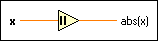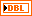# Absolute Value Function

## LabVIEW 2018 Help

Edition Date: March 2018
Part Number: 371361R-01
View Product Info

### DOWNLOAD (Windows Only)LabVIEW 2016 HelpLabVIEW 2017 HelpLabVIEW 2018 HelpLabVIEW 2019 HelpLabVIEW 2020 Help

Owning Palette: Numeric Functions

Requires: Base Development System

Returns the absolute value of the input.

The connector pane displays the default data types for this polymorphic function.x can be a scalar number, a fixed-point number, an array or cluster of numbers, an array of clusters of numbers, and so on. x cannot be an unsigned integer, because unsigned integers represent only non-negative integers.abs(x) is the absolute value of x. When x is of the form x = a + bi, that is, when x is complex, the following equation defines abs(x):## Absolute Value Details

When you wire matrix data as an input to this function, a VI that includes subVIs that work with the matrix data type replaces the function. The resulting VI has the same icon but contains a matrix-specific algorithm. The node remains a VI if you disconnect the matrix from the input(s). Wire other data types as inputs to restore the original function. If you wire a data type to a function and that data type causes a basic math operation to fail, the function returns an empty matrix or NaN.

## Example

Refer to the Numeric Functions VI in the labview\examples\Numerics directory for an example of using the Absolute Value function.

Not Helpful